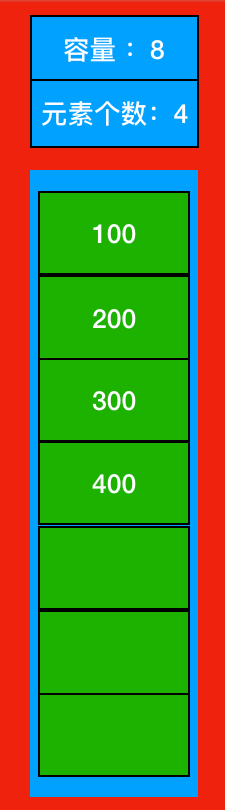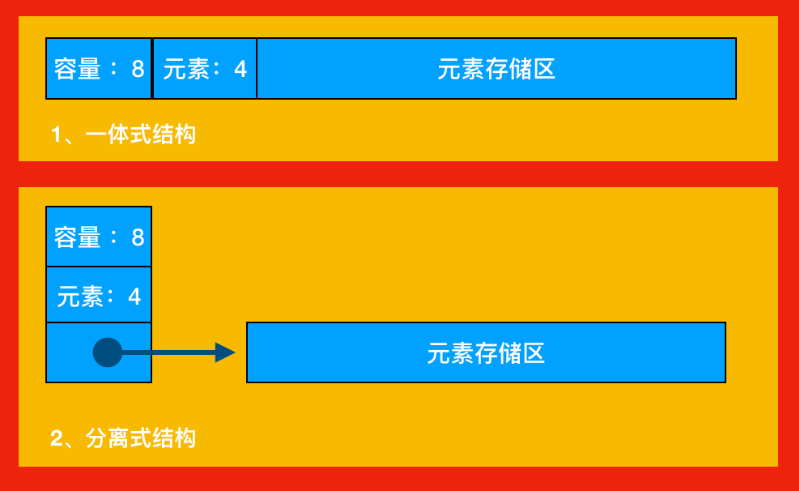Python中的顺序表是什么1、顺序表介绍上图1表示的是顺序表的基本形式，数据元素本身连续存储，每个元素所占的存储单元大小固定相同，元素的下标是其逻辑地址，而元素存储的物理地址（实际内存地址）可以通过存储区的起始地址Loc (e0)加上逻辑地址（第i个元素）与存储单元大小（c）的乘积计算而得，即：Loc(element i) = Loc(e0) + c*i

如果元素的大小不统一，则须采用图2的元素外置的形式，将实际数据元素另行存储，而顺序表中各单元位置保存对应元素的地址信息（即链接）。由于每个链接所需的存储量相同，通过上述公式，可以计算出元素链接的存储位置，而后顺着链接找到实际存储的数据元素。注意，图2中的c不再是数据元素的大小，而是存储一个链接地址所需的存储量，这个量通常很小。

2、顺序表的结构一个顺序表的完整信息包括两部分，一部分是表中的元素集合，另一部分是为实现正确操作而需记录的信息，即有关表的整体情况的信息，这部分信息主要包括元素存储区的容量和当前表中已有的元素个数两项。

3、顺序表的两种基本实现方式1为一体式结构，存储表信息的单元与元素存储区以连续的方式安排在一块存储区里，两部分数据的整体形成一个完整的顺序表对象。一体式结构整体性强，易于管理。但是由于数据元素存储区域是表对象的一部分，顺序表创建后，元素存储区就固定了。

2为分离式结构，表对象里只保存与整个表有关的信息（即容量和元素个数），实际数据元素存放在另一个独立的元素存储区里，通过链接与基本表对象关联。

4、元素存储区替换

一体式结构由于顺序表信息区与数据区连续存储在一起，所以若想更换数据区，则只能整体搬迁，即整个顺序表对象（指存储顺序表的结构信息的区域）改变了。分离式结构若想更换数据区，只需将表信息区中的数据区链接地址更新即可，而该顺序表对象不变。

5、元素存储区扩充

采用分离式结构的顺序表，若将数据区更换为存储空间更大的区域，则可以在不改变表对象的前提下对其数据存储区进行了扩充，所有使用这个表的地方都不必修改。只要程序的运行环境（计算机系统）还有空闲存储，这种表结构就不会因为满了而导致操作无法进行。人们把采用这种技术实现的顺序表称为动态顺序表，因为其容量可以在使用中动态变化。

6、顺序表的增删改查操作的Python代码实现

# 创建顺序表class Sequence_Table():
# 初始化
def __init__(self):
self.date = [None]*100
self.length = 0
# 判断是否已经满了
def isFull(self):
if self.length>100:
print("该顺序表已满，无法添加元素")
return 1
else:
return 0
# 按下表索引查找
def selectByIndex(self,index):
if index>=0 and index<=self.length-1:
return self.date[index]
else:
print("你输入的下标不对，请重新输入\n")
return 0
# 按元素查下标
def selectByNum(self,num):
isContain = 0
for i in range(0,self.length):
if self.date[i] == num:
isContain = 1
print("你要查找的元素下标是%d\n"%i)
if isContain == 0:
print("没有找你你要的数据")
# 追加数据
if self.isFull() == 0:
self.date[self.length] = num
self.length += 1
# 打印顺序表
def printAllNum(self):
for i in range(self.length):
print("a[%s]=%s"%(i,self.date[i]),end=" ")
print("\n")
# 按下标插入数据
def insertNumByIndex(self,num,index):
if index<0 or index>self.length:
return 0
self.length += 1
for i in range(self.length-1,index,-1):
temp = self.date[i]
self.date[i] = self.date[i-1]
self.date[i-1] = temp
self.date[index] = num
return 1    # 按下标删除数据
def delectNumByIndex(self,index):
if self.length <= 0:
print("该顺序表内没有数据，不用删除")
for i in range(index,self.length-1):
temp = self.date[i]
self.date[i] = self.date[i + 1]
self.date[i + 1] = temp
self.date[self.length-1] = 0
self.length -= 1def main():    # 创建顺序表对象
seq_t = Sequence_Table()
# 插入三个元素
# 打印验证
seq_t.printAllNum()
# 按照索引查找
num = seq_t.selectByIndex(2)
print("你要查找的数据是%d\n" % num)
# 按照索引插入数据
seq_t.insertNumByIndex(4, 1)
seq_t.printAllNum()
# 按照数字查下标
seq_t.selectByNum(4)
#删除数据
seq_t.delectNumByIndex(1)
seq_t.printAllNum()
if __name__ == "__main__":
main()

a=1 a=2 a=3

a=1 a=4 a=2 a=3

a=1 a=2 a=3

7、顺序表的增删改查操作的C语言代码实现

#include<stdio.h>
// 1、定义顺序表的储存结构
typedef struct
{
//用数组存储线性表中的元素
int data;
// 顺序表中的元素个数
int length;
}Sequence_table,*p_Sequence_table;
// 2、顺序表的初始化，
void initSequenceTable(p_Sequence_table T)
{
// 判断传过来的表是否为空，为空直接退出
if (T == NULL)
{
return;
}
// 设置默认长度为0
T->length = 0;
}
// 3、求顺序表的长度
int lengthOfSequenceTable(p_Sequence_table T)
{
if (T==NULL)
{
return 0;
}
return T->length;
}
// 4、判断顺序表是否已满
int isFull(p_Sequence_table T)
{
if (T->length>=100)
{
printf("该顺序表已经装满，无法再添加元素");
return 1;
}
return 0;
}
// 5、按序号查找
int selectSequenceTableByIndex(p_Sequence_table T,int index)
{
if (index>=0&&index<=T->length-1)
{
return T->data[index];
}
printf("你输入的序号不对，请重新输入\n");
return 0;
}
// 6、按内容查找是否存在
void selectSequenceTableByNum(p_Sequence_table T,int num)
{
int isContain = 0;
for (int i=0; i<T->length; i++)
{
if (T->data[i] == num)
{
isContain = 1;
printf("你要找的元素的下标是:%d\n",i);
}
}
if (isContain == 0)
{
printf("没有找到你要的数据\n");
}
}
// 7、添加元素（在队尾添加）
{
// 顺序表还没有满的时候
if (isFull(T) == 0)
{
T->data[T->length] = num;
T->length++;
}
}
// 8、顺序表的遍历
void printAllNumOfSequenceTable(p_Sequence_table T)
{
for (int i = 0; i<T->length; i++)
{
printf("T[%d]=%d ",i,T->data[i]);
}
printf("\n");
}
//9、插入操作
int insertNumByIndex(p_Sequence_table T, int num,int index)
{
if (index<0||index>T->length)
{
return 0;
}
T->length++;
for (int i = T->length-1; i>index; i--)
{
int temp = T->data[i];
T->data[i] = T->data[i-1];
T->data[i-1] = temp;
}
T->data[index] = num;
return 1;
}
// 10、删除元素
void delectNum(p_Sequence_table T,int index)
{
if (T->length <= 0)
{
printf("该顺序表中没有数据，不用删除");
}
for (int i = index;i<T->length-1; i++)
{
int temp = T->data[i];
T->data[i] = T->data[i+1];
T->data[i+1] = temp;
}
T->data[T->length-1] = 0;
T->length--;
}
int main(int argc, const char * argv[]) {

// 创建顺序表的结构体
Sequence_table seq_t;
// 初始化
initSequenceTable(&seq_t);
// 添加数据
// 打印验证
printAllNumOfSequenceTable(&seq_t);
// 根据索引下标查内容
int num = selectSequenceTableByIndex(&seq_t, 2);
printf("你查的数据是:%d\n",num);
// 插入
insertNumByIndex(&seq_t, 4, 1);
printAllNumOfSequenceTable(&seq_t);
// 根据内容查下标
selectSequenceTableByNum(&seq_t, 4);
// 根据下标删除数据
delectNum(&seq_t, 1);
printAllNumOfSequenceTable(&seq_t);
return 0;
}

T=1 T=2 T=3

T=1 T=4 T=2 T=3

T=1 T=2 T=3

Python开发开发项目